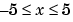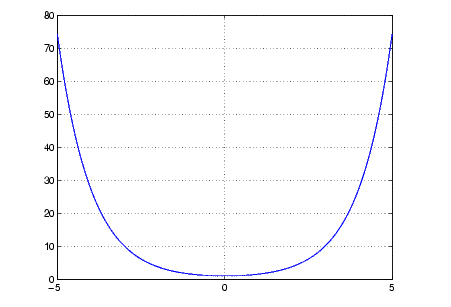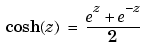MATLAB Function Referencecosh

Hyperbolic cosine

Syntax

• ```Y = cosh(X)
```

Description

The `cosh` function operates element-wise on arrays. The function's domains and ranges include complex values. All angles are in radians.

```Y = cosh(X) ``` returns the hyperbolic cosine for each element of `X`.

Examples

Graph the hyperbolic cosine function over the domain.

• ```x = -5:0.01:5;
plot(x,cosh(x)), grid on```

Definition

The hyperbolic cosine can be defined as

•Algorithm

`cosh` uses FDLIBM, which was developed at SunSoft, a Sun Microsystems, Inc. business, by Kwok C. Ng, and others. For information about FDLIBM, see http://www.netlib.org.

See Also

`acos`, `acosh`, `cos`

© 1994-2005 The MathWorks, Inc.